# 灰色预测模型

## 灰色预测的概念

### （1）灰色系统、白色系统和黑色系统

• 白色系统是指一个系统的内部特征是完全已知的，既系统信息是完全充分的。
• 黑色系统是一个系统的内部信息对外界来说是一无所知的，只能通过它与外界的联系来加以观测研究。
• 灰色系统介于白色和黑色之间，灰色系统内的一部分信息是已知的，另一部分信息是未知的，系统内各因素间有不确定的关系。

### （2）灰色预测法

• 灰色预测法是一种预测灰色系统的预测方法。
• 灰色预测通过鉴别系统因素之间发展趋势的相异程度，即进行关联分析，并对原始数据进行生成处理来寻找系统变动的规律，生成有较强规律性的数据序列，然后建立相应的微分方程模型，从而预测事物未来发展趋势的状况。

## 关联分析

关联分析实际上是动态过程发展态势的量化比较分析。所谓发展态势比较，也就是系统各时期有关统计数据的集合关系的比较。

例如，某地区1977~1983年总收入与养猪、养兔收入资料见下表格。

1977 1978 1979 1980 1981 1982 1983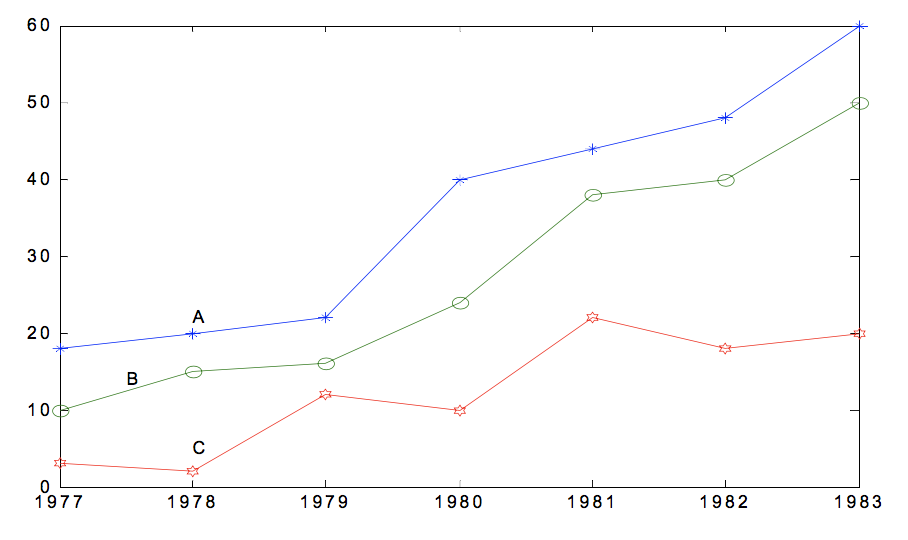由上图易看出，曲线 A(总收入)与曲线 B(养猪收入)发展趋势比较接近，而与 曲线 C(养兔收入)相差较大，因此可以判断，该地区对总收入影响较直接的是养猪业， 而不是养兔业。

很显然，几何形状越接近，关联程度也就越大。当然，直观分析对于稍微复杂些 的问题则显得难于进行。因此，需要给出一种计算方法来衡量因素间关联程度的大小。

## 灰色生成数列

灰色系统理论认为，尽管客观表象复杂，但总是有整体功能的，因此必然蕴含某种内在规律。关键在于如何选择适当的方式去挖掘和利用它。灰色系统时通过对原始数据的整理来寻求其变化规律的，这是一种就数据寻求数据的现实规律的途径，也就是灰色序列的生产。一切灰色序列都能通过某种生成弱化其随机性，显现其规律性。数据生成的常用方式有累加生成累减生成加权累加生成

### （1）累加生成（AGO）

设原始数列为x(0)=(x0(1),x0(2),.x0(n))$x^{(0)}= (x^{0}(1),x^{0}(2),….x^{0}(n))$，令

x(1)(k)=i=1kx(0)(i),k=1,2,...,n

x(1)=(x1(1),x1(2),.x1(n))

称所得到的新数列为数列x(0)$x^{(0)}$的1次累加生成数列。类似的有

x(r)(k)=i=1kx(r1)(i),k=1,2,...,n,r1

称为x(0)$x^{(0)}$的r次累加生成数列。

### （2）累减生成(IAGO)

如果原始数列为x(1)=(x1(1),x1(2),.x1(n))$x^{(1)}= (x^{1}(1),x^{1}(2),….x^{1}(n))$，令

x(0)(k)=x(1)(k)x(1)(k1),k=2,3,...,n

称所得到的数列x(0)$x^{(0)}$x(1)$x^{(1)}$的1次累减生成数列。 可以看出，通过累加数列的到的新数列，可以通过累减生成还原出原始数列。

### （3）加权邻值生成

如果原始数列为x(1)=(x1(1),x1(2),.x1(n))$x^{(1)}= (x^{1}(1),x^{1}(2),….x^{1}(n))$，称任意一对相邻元素x(0)(k1),x(0)(k)$x^{(0)}(k-1),x^{(0)}(k)$互为邻值。对于常数α[0,1]$\alpha\in[0,1]$，令

z(0)(k)=αx(0)(k)+(1α)x(0)(k1),k=2,3,,n

由此得到的数列称为邻值生成数，权α$\alpha$也称为生成系数。 特别地，当生成系数α=0.5$\alpha = 0.5$时，则称该数列为均值生成数，也称为等权邻值生成数。

### 累加生成的特点

一般经济数列都是非负数列。累加生成能使任意非负数列、摆动的与非摆动的，转化为非减的、递增的。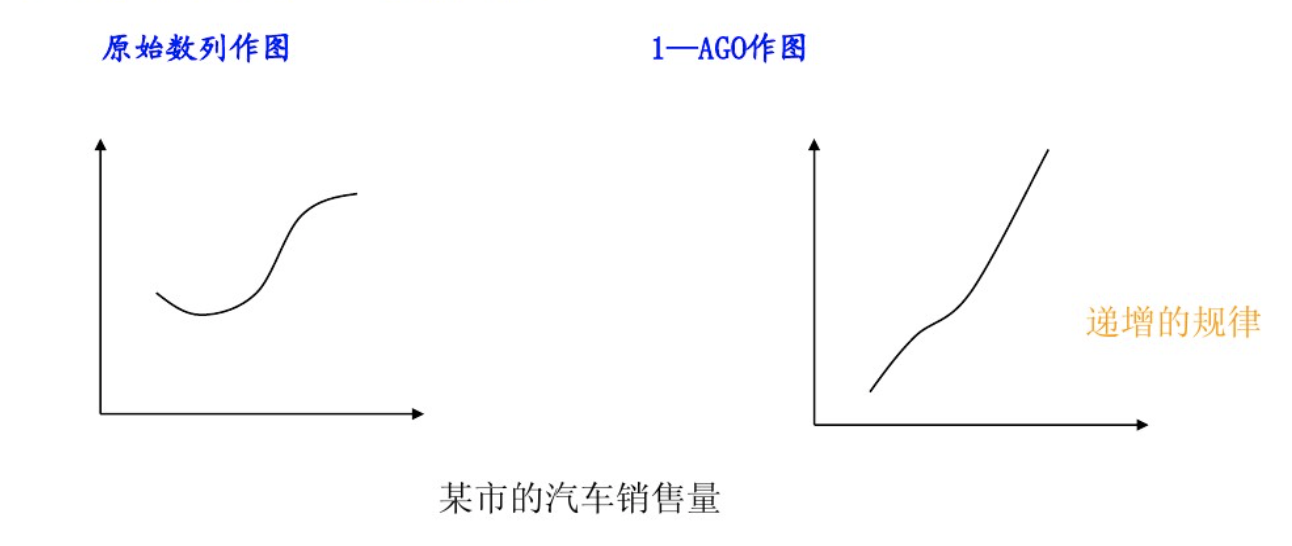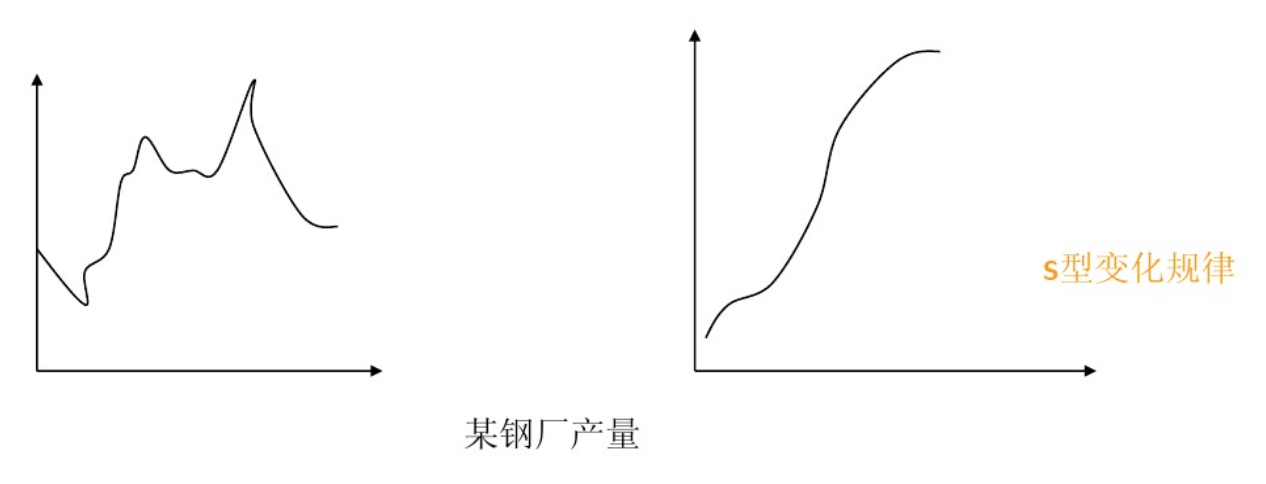## 灰色模型GM(1,1)

灰色系统理论是基于关联空间、光滑离散函数等概念定义灰导数与灰微分方程，进而用离散数据列建立微分方程形式的动态模型，即灰色模型是利用离散随机数经过生成变为随机性被显著削弱而且较有规律的生成数，建立起的微分方程形式的模型，这样便于对其变化过程进行研究和描述。

G表示grey（灰色），M表示model（模型）

定义x(1)$x^{(1)}$的灰导数为

d(k)=x(0)(k)=x(1)(k)x(1)(k1)

令z(1)(k)$z^{(1)}(k)$为数列x(1)$x^{(1)}$的邻值生成数列，即
z(1)(k)=αx(1)(k)+(1α)x(1)

于是定义GM（1,1）的灰微分方程模型为
d(k)+αz(1)(k)=bx(0)(k)+αz(1)(k)=b

其中，x(0)(k)$x^{(0)}(k)$称为灰导数，α$\alpha$称为发展系数，z(1)(k)$z^{(1)}(k)$称为白化背景值，b称为灰作用量。

将时刻k=2,3,…,n代入上式有

x(0)(2)+αz(1)(2)=bx(0)(3)+αz(1)(3)=b...x(0)(n)+αz(1)(n)=b

引入矩阵向量记号：
u=[ab]Y=x(0)(2)x(0)(2)...x(0)(n)B=z(1)(2)z(1)(3)...z(1)(n)11...1

于是GM(1,1)模型可表示为Y=Bu$Y=Bu$.

那么现在的问题就是求a和b的值，我们可以用一元线性回归，也就是最小二乘法求它们的估计值为

u=[ab]=(BTB)1BTY

## GM(1,1)的白化型

对于GM（1，1）的灰微分方程，如果将时刻k=2,3,…,n视为连续变量t，则之前的x(1)$x^{(1)}$视为时间t函数，于是灰导数x(0)(k)$x^{(0)}(k)$变为连续函数的导数dx(1)(t)dt$\frac{dx^{(1)}(t)}{dt}$,白化背景值z(1)(k)$z{(1)}(k)$对应于导数x(1)(t)$x^{(1)}(t)$。于是GM（1，1）的灰微分方程对应于的白微分方程为：

dx(1)(t)dt+ax(1)(t)=b

## GM（1，1）灰色预测的步骤

### 1.数据的检验与处理

为了保证GM（1，1）建模方法的可行性，需要对已知数据做必要的检验处理。

设原始数据列为x(0)=(x0(1),x0(2),.x0(n))$x^{(0)}= (x^{0}(1),x^{0}(2),….x^{0}(n))$，计算数列的级比

λ(k)=x(0)(k1)x(0)(k),k=2,3,...,n

如果所有的级比都落在可容覆盖区间X=(e2n+1,e2n+1)$X=(e^{\frac{-2}{n+1}},e^{\frac{2}{n+1}})$内，则数列x(0)$x^{(0)}$可以建立GM（1，1）模型且可以进行灰色预测。否则，对数据做适当的变换处理，如平移变换：
y(0)(k)=x(0)(k)+c,k=1,2,...,n

取c使得数据列的级比都落在可容覆盖内。

### 2.建立GM（1，1）模型

不妨设x(0)=(x0(1),x0(2),.x0(n))$x^{(0)}= (x^{0}(1),x^{0}(2),….x^{0}(n))$满足上面的要求，以它为数据列建立GM（1，1）模型

x(0)(k)+αz(1)(k)=b

用回归分析求得a，b的估计值，于是相应的白化模型为
dx(1)(t)dt+ax(1)(t)=b

解为
x(1)(t)=(x(0)(1)ba)ea(t1)+ba

于是得到预测值
x̂ (1)(k+1)=(x(0)(1)ba)eak+ba,k=1,2,...,n1

从而相应地得到预测值：
x̂ (0)(k+1)=x̂ (1)(k+1)x̂ (1)(k),k=1,2,...,n1

### 3.检验预测值

#### （1）残差检验：计算相对残差

ε(k)=x(0)(k)x̂ (0)(k)x(0)(k),k=1,2,...,n

如果对所有的|ε(k)|<0.1$|\varepsilon(k)|<0.1$，则认为到达较高的要求；否则，若对所有的|ε(k)|<0.2$|\varepsilon(k)|<0.2$，则认为达到一般要求。

#### （2）级比偏差值检验：计算

ρ(k)=110.5a1+0.5aλ(k)

如果对所有的|ρ(k)|<0.1$|\rho(k)|<0.1$，则认为达到较高的要求；否则，若对于所有的|ρ(k)|<0.2$|\rho(k)|<0.2$,则认为达到一般要求。

## 灰色预测计算实例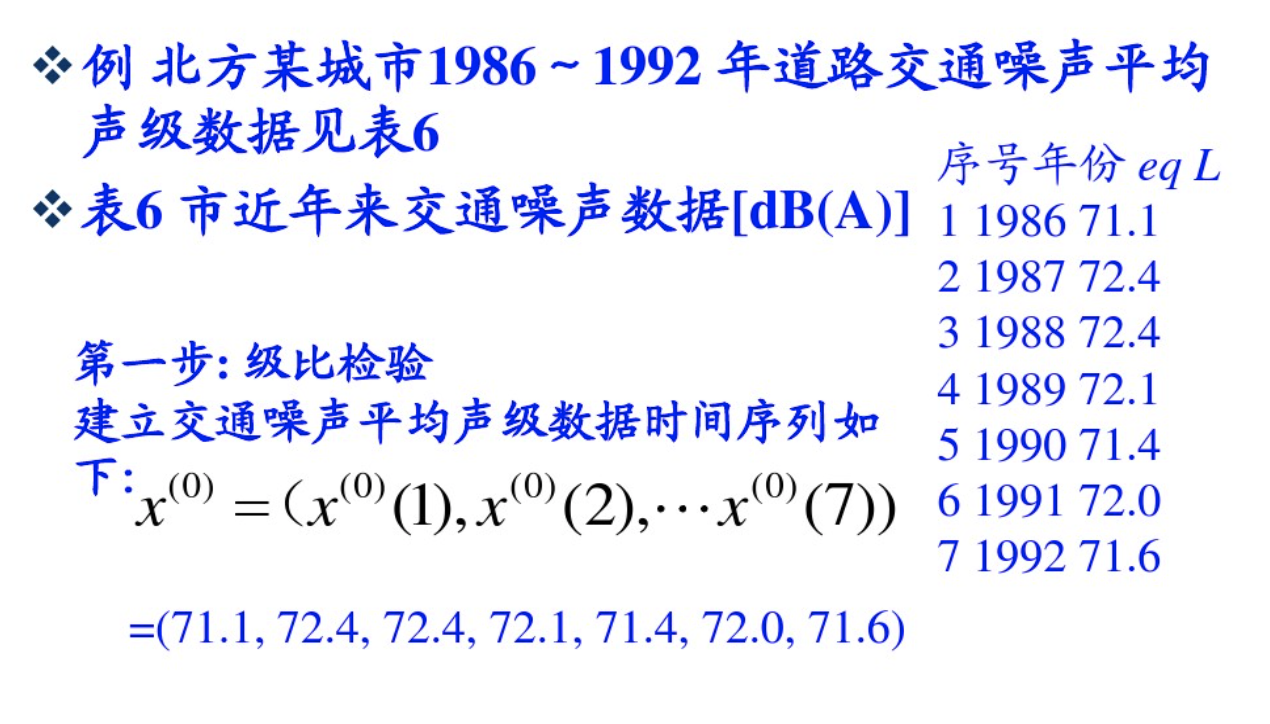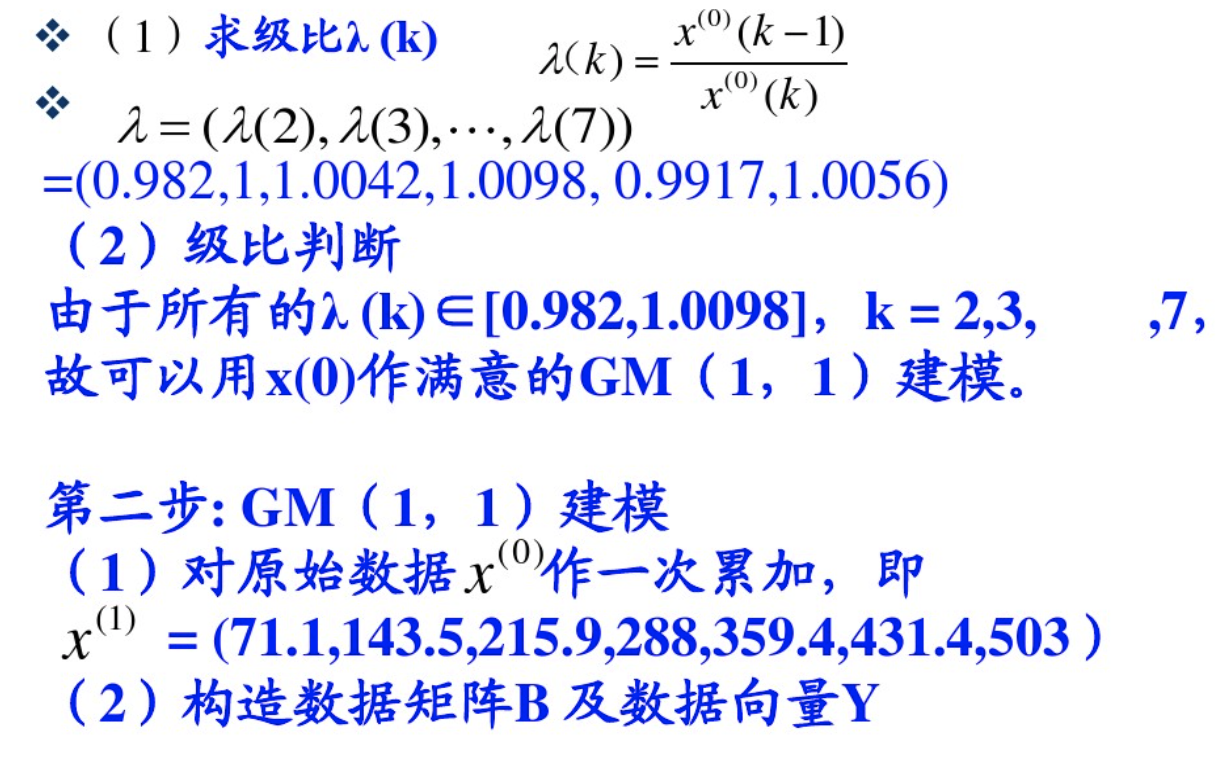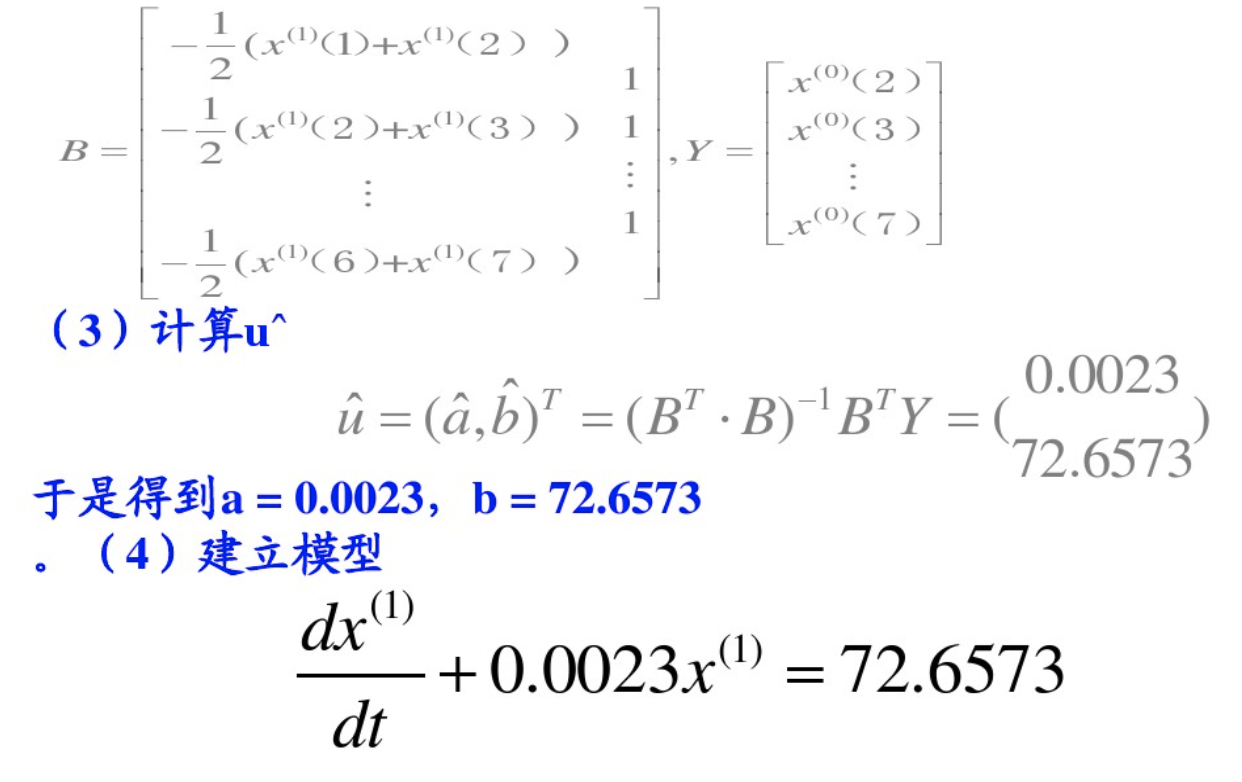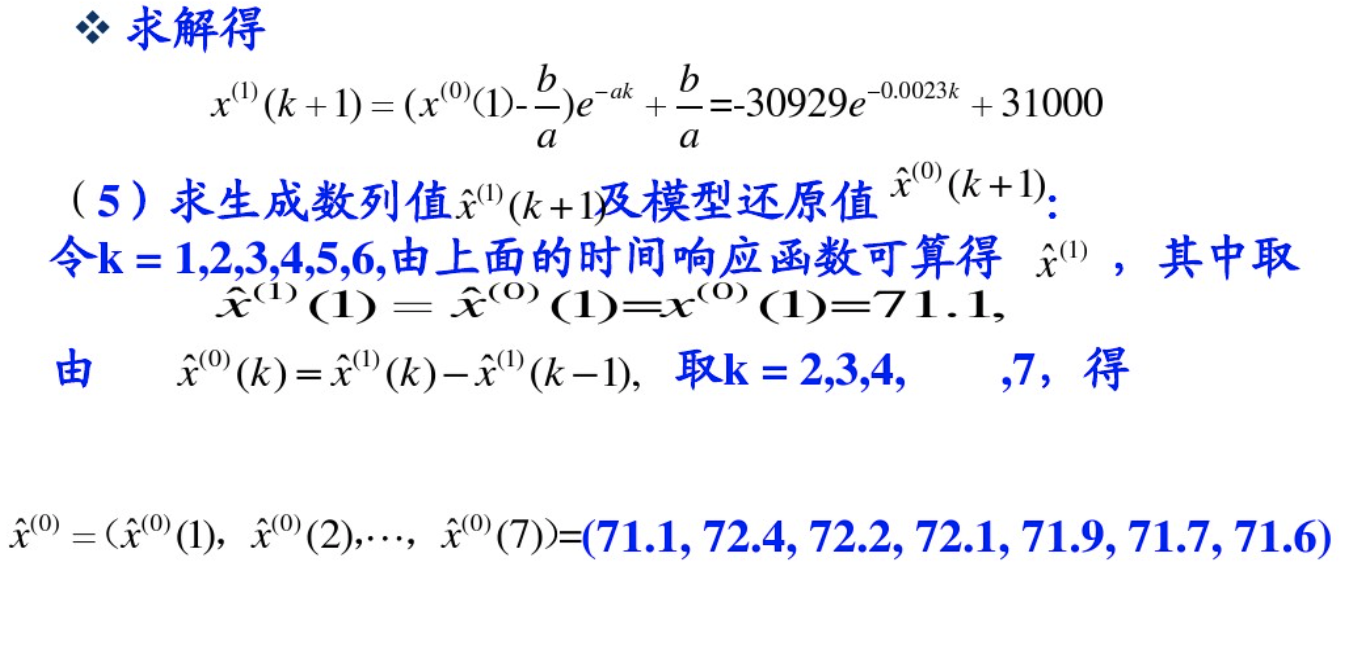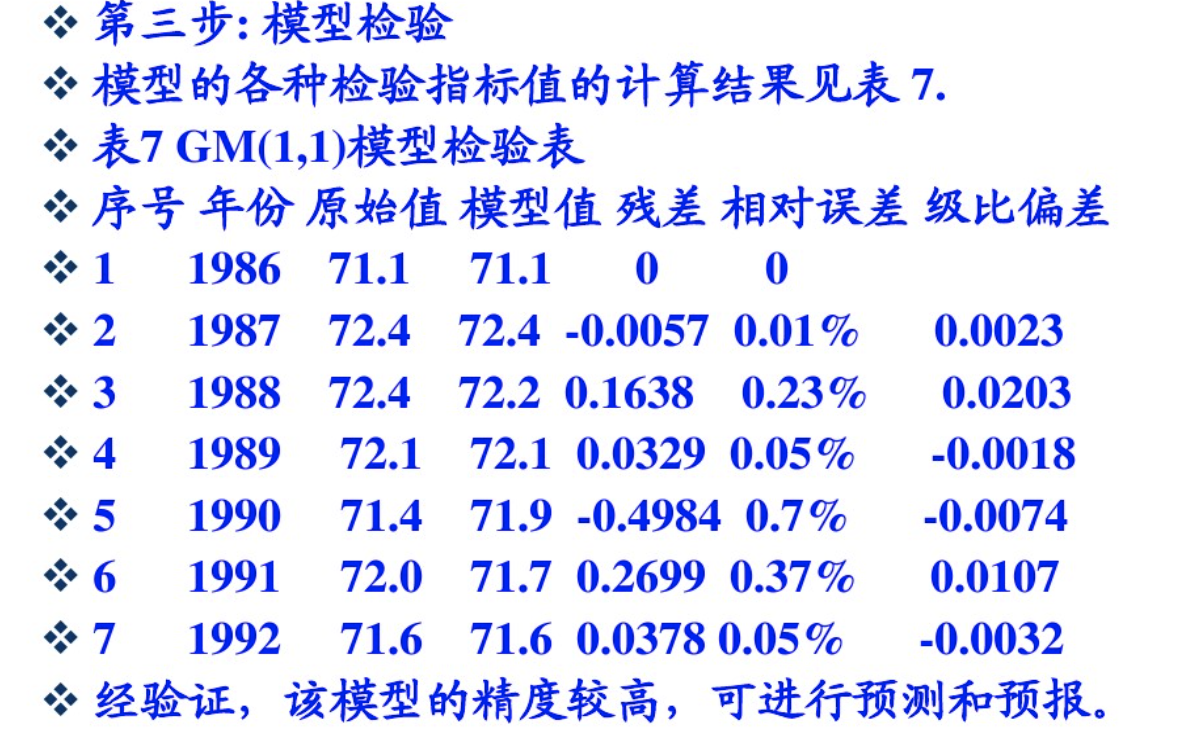©️2019 CSDN 皮肤主题: 撸撸猫 设计师: 设计师小姐姐